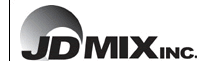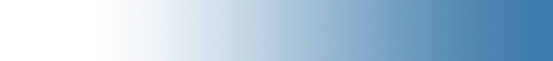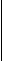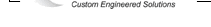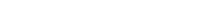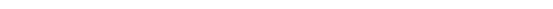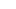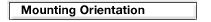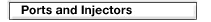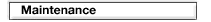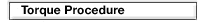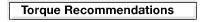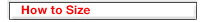How to Size your JDMIX Static Mixer
for turbulent flow

Sizing a typical JDMIX motionless mixer for water like applications is very simple. The formulas listed below for Reynolds number and velocity will enable you to determine just how many mixing elements are necessary for your application.

1) Calculate the Reynolds number for your application to make sure you are in turbulent flow

Re = 3157QS/uD

 Q = flow rate in gallons per minute S = Specific Gravity u = Viscosity D = Inside diameter of your pipeline in inches

Example: 100 gpm of water flowing in a 3 inch carbon steel pipeline

 Q = 100 gpm S = 1.0 u = 1.0 D = 3.068

Reynolds Number = 102,900

2) Calculate the velocity for your application in fps

V = .408Q/d2 = fps

 Q = flow rate in gallons per minute d = Inside diameter of your pipeline in inches

Example: 100 gpm of water flowing in a 3 inch carbon steel pipeline

 Q = 100 gpm d = 3.068

Velocity = 4.33 fps

If you are in turbulent flow, the number of mixing elements necessary for 100% homogeneous mixing is as follows:

 Velocity Range Number of Mixing Elements Required 0 - 1 fps 6 elements 1 - 3 fps 5 elements 3 - 4 fps 4 elements 4 - 7 fps 3 elements 8 - 10 fps 2 elements

For the example above, going 4.3 fps in a 3 inch diameter pipeline, you need three mixing elements for a 100% homogeneous mix.